Related Articles
Categorical Representation of Data in Julia
• Last Updated : 25 Aug, 2020

Julia is a high performance, dynamic programming language that is easy to learn as it is a high-level language. But sometimes, when dealing with data in programming languages like Julia, we encounter structures or representations with a small number of levels as represented below.

## Julia

 `# Creating an array of stings``a ``=` `[``"Geeks"``, ``"For"``, ``"Geeks"``, ``"Useful"``, ``"For"``, ``"Everybody"``]`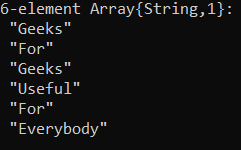As you can see, the elements of the array are simply categorized as full strings.

#### Categorical Data

By changing the array type to CategoricalArray type we can represent the elements better to make things easier in the future for some tasks. The CategoricalArray type represents the strings as indices in a number of levels.

## Julia

 `# Creating an array of the ``# CategoricalArray type of the array 'a'``cat ``=` `CategoricalArray(a)`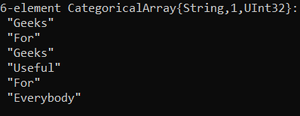In the example mentioned above, 232 levels are represented (UInt32).

CategoricalArray type can also classify a missing value as shown below:

## Julia

 `# Creating array of CategoricalArray ``# type with some missing values``cat ``=` `CategoricalArray([``"Geeks"``, ``"For"``, ``"Geeks"``,``                         ``missing, missing, ``"Everybody"``])`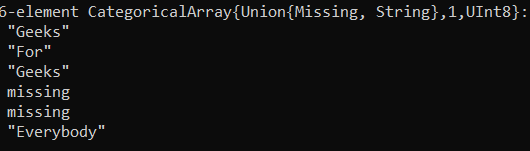#### Levels of the Array

CategoricalArray type allows us to know the levels which are valid as there are repeated data, by using the levels() function where the argument to be passed is the array.

## Julia

 `# Determining levels of the array``levels(cat)`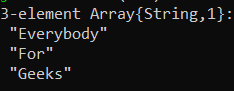We can change the placement or order of the levels by using the levels!() function, as it might be useful later on.

## Julia

 `# Changing the order of the levels displayed``levels!(cat, [``"Geeks"``, ``"For"``, ``"Everybody"``]);``levels(cat)`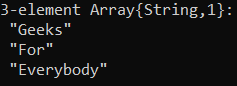And we can sort the array according to the changed order of the levels.

## Julia

 `# Sorting array according to the levels``sort(cat)`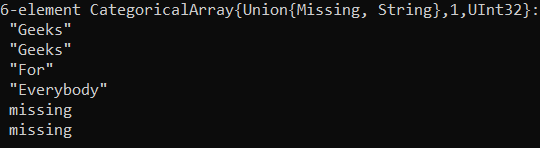#### Compression of levels

The CategoricalArray type can have 232 levels as shown in the description of the array in the outputs. If these many levels are not required we decrease them by using the compress() function. The following example shows the decrease of the levels to 28 levels.

## Julia

 `# Decreasing the number of levels for the array 'cat'``cat ``=` `compress(cat)`#### Categorical function

We can directly use the categorical function instead of using CategoryArrays which allows us to apply a keyword argument like the compress keyword which when set to ‘true’, implicates implementation of that keyword on the elements.

## Julia

 `# Creating a categorical array and ``# applying the compress function``cat2 ``=` `categorical([``"Geeks"``, ``"For"``, ``"Geeks"``], compress ``=` `true)`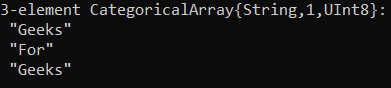In the same way, we have implemented the compress keyword, the ordered keyword can be implemented by equating it to ‘true’, which gives an order to the levels of the array.

## Julia

 `# Creating an ordered categorical array``cat3 ``=` `categorical([``"Geeks"``, ``"For"``, ``"Geeks"``], ordered``=``true)`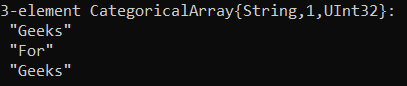#### Order of the levels

We can check the levels of arrays for order and when it is not an ordered array, it produces an error as shown below.

## Julia

 `# Testing levels of unordered array``cat2[``1``] < cat2[``2``]`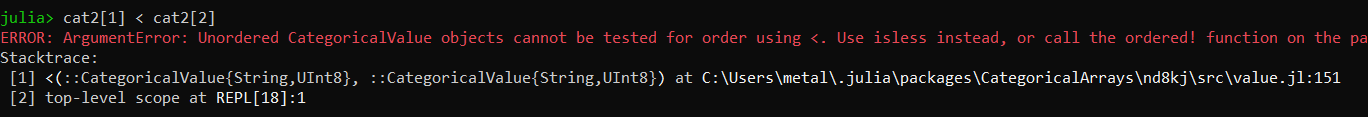When the array is ordered, it results in either true or false based on the order of the levels.

## Julia

 `# Tesing levels of the ordered array``cat3[``1``] < cat3[``2``]`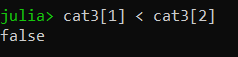We can check whether if an array is ordered with the isordered() function.

## Julia

 `# Checking if array is ordered``isordered(cat2)`We can change an unordered array to ordered and vice-versa by using the ordered!() function.

## Julia

 `# Changing unordered array to an ordered array``ordered!(cat2, true)`

Now that we have ordered the array, we can test it.

## Julia

 `# Testing levels of the array``cat2[``1``] < cat2[``2``]`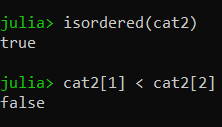#### Categorical data in a DataFrame

We can implement the categorical function on one or more columns of a Dataframe by using the categorical!() function in which the first argument is the DataFrame and the second argument can be columns of the DataFrame we want to apply on and some keyword function.

## Julia

 `# Creating a DataFrame with String elements``using DataFrames`` ` `df ``=` `DataFrame(A ``=` `[``"A"``, ``"A"``, ``"A"``, ``"B"``, ``"B"``, ``"C"``],``               ``B ``=` `[``"D"``, ``"E"``, ``"E"``, ``"F"``, ``"G"``, ``"G"``])`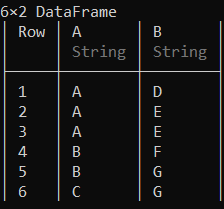We can change the type of a specific column of the DataFrame to categorical type.

## Julia

 `# Changing the column A to categorical``categorical!(df, :A)`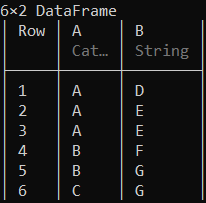If we don’t specify the column, the columns with an AbstractString type will change to categorical. By equating compress keyword function to true we can apply the function on all of the columns.

## Julia

 `# Changing columns to categorical type``categorical!(df, compress``=``true)`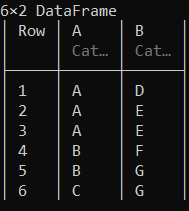We can check the types of the columns of the DataFrame with eltype() function.

## Julia

 `# Displaying column types``eltype.(eachcol(df))`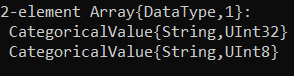My Personal Notes arrow_drop_up